# Related Videos

## Factor the expression $x^2-2xy+y^2$

Go!
1
2
3
4
5
6
7
8
9
0
a
b
c
d
f
g
m
n
u
v
w
x
y
z
.
(◻)
+
-
×
◻/◻
/
÷
2

e
π
ln
log
log
lim
d/dx
Dx
|◻|
θ
=
>
<
>=
<=
sin
cos
tan
cot
sec
csc

asin
acos
atan
acot
asec
acsc

sinh
cosh
tanh
coth
sech
csch

asinh
acosh
atanh
acoth
asech
acsch

### Videos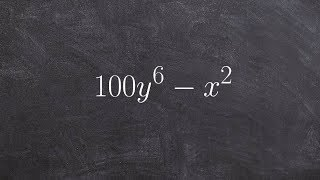### Algebra 1 - Factor difference of two squares - The best math teacher ever 100y^6 - x^2

https://www.youtube.com/watch?v=0mjt0uzT73c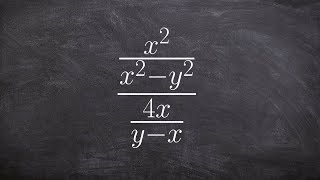### Algebra 2 - Dividing two rational expressions (x^2/x^2 - y^2) / (4x/y-x)

https://www.youtube.com/watch?v=5MNXHwO_COA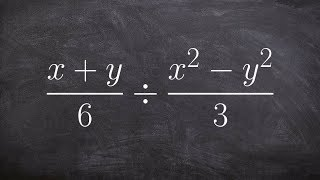### Algebra 2 - Dividing two rational expressions by factoring, ((x + y)/6) / ((x^2 - y^2)/3)

https://www.youtube.com/watch?v=mAn_OHWNj2c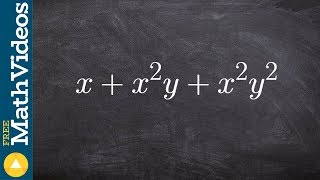### Algebra 1 - How to factor the GCF from a trinomial x+ x^2y + x^2 y^2

https://www.youtube.com/watch?v=-uxboTtK1jg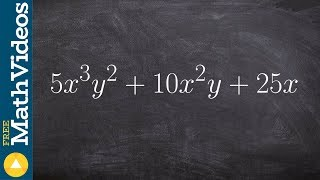### Algebra 1 - Factoring three terms with distributive property 5x^3 y^2 + 10x^2y + 25x

https://www.youtube.com/watch?v=n2ymRq-teXc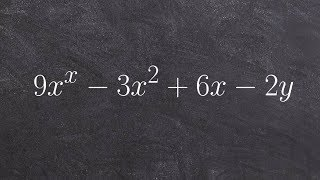### Tutorial - Factor an expression by using grouping, 9x^2 - 3xy + 6x - 2y

https://www.youtube.com/watch?v=mN3WP4KGxz0
SnapXam A2
Answer Assistant

1
2
3
4
5
6
7
8
9
0
a
b
c
d
f
g
m
n
u
v
w
x
y
z
.
(◻)
+
-
×
◻/◻
/
÷
2

e
π
ln
log
log
lim
d/dx
Dx
|◻|
θ
=
>
<
>=
<=
sin
cos
tan
cot
sec
csc

asin
acos
atan
acot
asec
acsc

sinh
cosh
tanh
coth
sech
csch

asinh
acosh
atanh
acoth
asech
acsch

### Tips on how to improve your answer:

$factor\left(x^2-2xy+y^2\right)$

Factorization

~ 0.26 s